Zhou, Q., Cai, Y., Xu, Y., and Zhang, X. (2011). "Determination of moisture diffusion coefficient of larch board with finite difference method," BioRes. 6(2), 1196-1203.

#### Abstract

This paper deals with the moisture diffusion coefficient of Dahurian Larch (Larix gmelinii Rupr.) by use of the Finite Difference Method (FDM). To obtain moisture distributions the dimensional boards of Dahurian Larch were dried, from which test samples were cut and sliced evenly into 9 pieces in different drying periods, so that moisture distributions at different locations and times across the thickness of Dahurian Larch were obtained with a weighing method. With these experimental data, FDM was used to solve Fick’s one-dimensional unsteady-state diffusion equation, and the moisture diffusion coefficient across the thickness at specified time was obtained. Results indicated that the moisture diffusion coefficient decreased from the surface to the center of the Dahurian Larch wood, and it decreased with decreasing moisture content at constant wood temperature; as the wood temperature increased, the moisture diffusion coefficient increased, and the effect of the wood temperature on the moisture diffusion coefficient was more significant than that of moisture content. Moisture diffusion coefficients were different for the two experiments due to differing diffusivity of the specimens.

DETERMINATION OF MOISTURE DIFFUSION COEFFICIENT OF LARCH BOARD WITH FINITE DIFFERENCE METHOD

Qiaofang Zhou,a Yingchun Cai,a* Yan Xu a and Xiangling Zhang a

This paper deals with the moisture diffusion coefficient of Dahurian Larch (Larix gmelinii Rupr.) by use of the Finite Difference Method (FDM). To obtain moisture distributions the dimensional boards of Dahurian Larch were dried, from which test samples were cut and sliced evenly into 9 pieces in different drying periods, so that moisture distributions at different locations and times across the thickness of Dahurian Larch were obtained with a weighing method. With these experimental data, FDM was used to solve Fick’s one-dimensional unsteady-state diffusion equation, and the moisture diffusion coefficient across the thickness at specified time was obtained. Results indicated that the moisture diffusion coefficient decreased from the surface to the center of the Dahurian Larch wood, and it decreased with decreasing moisture content at constant wood temperature; as the wood temperature increased, the moisture diffusion coefficient increased, and the effect of the wood temperature on the moisture diffusion coefficient was more significant than that of moisture content. Moisture diffusion coefficients were different for the two experiments due to differing diffusivity of the specimens.

Key words: Finite Difference Method; Unsteady-state diffusion; Moisture diffusion coefficient; Dahurian Larch board

Contact information: a: Key Laboratory of Bio-based Material Science and Technology of Ministry of Education, Northeast Forestry University, 150040, Harbin, China; *Corresponding author: ychcai@yahoo.com.cn

INTRODUCTION

The rate of bound water movement in wood is determined by a crucial factor, namely, the moisture diffusion coefficient. Skaar (1954) initially introduced Newman’s general solution (Newman 1931) of the one-dimensional unsteady-state diffusion equation into the wood drying field and solved the moisture diffusion coefficient successfully. Choong and Skaar (1969) invented a method in which two samples with different thickness were used to calculate the moisture diffusion coefficient and the surface emission coefficient at Ē=0.5. Avramidis and Siau (1987) investigated the effect of specimen thickness, moisture content, and temperature on the moisture diffusion and surface emission coefficient by using the empirical relationship between moisture diffusion coefficient and surface emission coefficient fitted by Choong and Skaar (1969). Liu (1989) developed a method from an analytical procedure to separate the moisture diffusion and surface emission coefficients in Newman’s general solution of the unsteady-state equation. His method required knowledge of a single drying curve such as the drying time and the slope of that curve at Ē=0.5. Liu and Simpson (1999) used two-stage approaches to solve the moisture diffusion coefficient and surface emission coefficient of the northern red oak. Their conclusion was that the transport coefficients for the northern red oak were not constant.

Liu et al. (2001) attempted to determine the moisture diffusion of northern red oak with the Finite Difference Method (FDM). In their approach the functional form of the diffusion coefficient as well as the boundary conditions at the surfaces were not known a priori, but special care had to be taken due to the sensitivity of the solution. Their results demonstrated that FDM could be a successful solution for the determination of moisture diffusion coefficient. Due to its advantages, the present research used FDM to determine the moisture diffusion coefficient of Dahurian Larch after gaining moisture content distributions through experiments carried out with convective drying. To the author’s knowledge, this might be the first attempt to determine the moisture diffusion coefficient of thick dimensional board in different period during convective drying, with analysis of the effect of moisture content and temperature on moisture diffusion coefficient. Since wood drying is essentially a process of heat and moisture transfer, the moisture diffusion coefficient, if determined correctly, could be later used as a physical parameter for the prediction of the moisture content variation in wood drying.

MATERIALS AND EXPERIMENT

Six specimens of 600 mm length were made from a dimensional board of Dahurian Larch (Larix gmelinii Rupr.) that was free of any visual defects and had about 30% initial moisture content. Each specimen was processed to the same thickness of 50 mm. The specimens were ends and edges coated with enough epoxy to guarantee moisture moved across the thickness of the specimens.

This experiment was conducted in a DS-408 conditioning chamber, where specimens were dried at different temperature and relative humidity. The velocity was about 2.0 m/s. The drying schedule for the Dahurian Larch was as follows: In the first period, dry-bulb temperature (Td) was 65 oC and wet-bulb temperature (Tw) 60 oC; in the second period, Td = 70 oC, and Tw = 62 oC; in the third period, Td =75 oC, and Tw =61 oC. During drying every 12h or 24h a specimen was taken out, and a moisture content sample was cut and quickly sliced evenly into 9 pieces. By this means the moisture distribution at different locations across the thickness was measured by a gravimetric method, at a specified time. If one sample was finished, another one was then used, and after cutting, the end was coated with epoxy again. Two runs of experimentation were conducted under the same conditions.

FINITE DIFFERENCE METHOD

As moisture moved across the thickness of the specimen, Fick’s one-dimensional unsteady-state diffusion equation was applied. The main assumptions used to formulate the model are listed below:

1. Moisture content and moisture diffusion across the thickness of the specimen are symmetrical, and the centerline of the specimen would be the moisture-insulated line; as a result only half of the specimen needs to be taken into account.

2. The boundary condition of the surface of the specimen needs not to be known a priori.

3. No chemical reaction or shrinking occurs during wood drying.

4. The moisture diffusion coefficient is a function of space and time.

5. For each period of the drying process, the temperature and relative humidity are constant. Since the speed of thermal diffusion in wood is 103 as fast as that of moisture diffusion, each period of the drying process is treated as an isothermal diffusion process.

Based on the above assumptions, the diffusion equation can be written as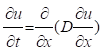, 0<x<,t>0 (1)

where u is moisture content, t is time, D is moisture diffusion coefficient, x is space coordinate measured from the surface of the specimen, and a is half of the thickness of the specimen. The initial condition can be stated as follows:

u=u(x, 0) (2)

The initial moisture distribution was determined from the experiment. Most earlier researchers have assumed that the moisture content was uniform at t=0; however, this is physically impossible in typical cases.

The boundary condition was assumed as follows: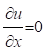, x=at>0 (3)

Let half of the specimen thickness be discretized with mesh width Δx in space and Δt in the time direction with grid points xj=j·Δx (where j=0,1,…, J) and ti=i·Δt (where i=0,1,…, I). FDM is applied here to write the above diffusion equation out a series of linear equations.

1. At any internal grid point 0<j<J and i≥0,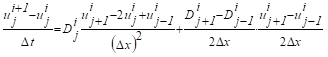(4)

Incorporating the same parameter yields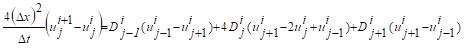(5)

2. At the surface grid point j=0 and i≥0,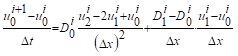(6)

Incorporating the same parameter yields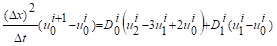(7)

3. At the center grid point j=J and i≥0, in term of assumption 1 there will be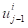=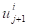，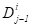=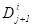，and setting these into equation (5) yields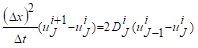(8)

In order to solve the linear equation, we set d=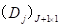, b=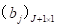, and A=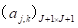. Matrix d represents the moisture diffusion coefficient D at discrete locations. Obviously, Dcan be obtained by solving the matrix equation Ad=b. Here the solution is d=A-1b. Elements of matrix b and A, not including zero elements, are as follows:

b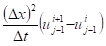，j=1, J+1 (9)

b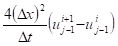，1<j<J+1 (10)

a1,1=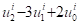(11)

a1,2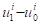(12)

ajj-1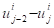, 1<j<J+1 (13)

aj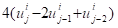, 1<j<J+1 (14)

ajj+1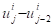, 1<j<J+1 (15)

aJ+1,J+1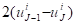(16)

The matrix equation can be solved without difficulty using Matlab software.

NUMERICAL RESULTS AND DISCUSSION

By use of the slicing method, moisture distributions were obtained at discrete points of x=2.78, 8.33, 13.89, 19.44, 23.61mm at specified times during drying. To reduce the effects of data scatter, the data were simulated by mathematical modeling. For example, data in the second period (Td =70 oC) of experiment 2 was fitted by a curve as shown in Fig. 1, which presents the variation of moisture content with space at t=28h. Variation of moisture content with time at x=7.5mm in the same period is presented in Fig. 2.

With this procedure moisture distributions across the thickness at specified times were estimated approximately. In the calculations with FDM, Δx andΔt were set 2.5 mm and 0.1 h, respectively.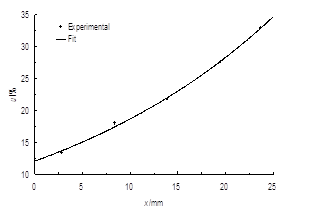u=-2.3167+14.3747exp(0.0378*x) Fig. 1. Moisture content as a function of space by curve fitting at t=28h of experiment 2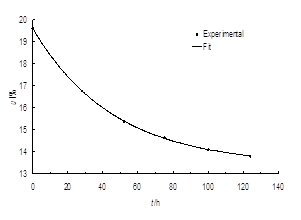u=13.409+6.1833exp(-0.0218*t) Fig. 2. Moisture content as a function of time by curve fitting at x=7.5mm of experiment 2

Variations of moisture diffusion coefficient as a function of space at specified time in the second period (Td =70 oC) of two drying processes are presented in Figs. 3 and 4, respectively. Neglecting the little increase at x=7.5 mm when t≤24h, moisture diffusion coefficient tended to decrease from the surface to the center at Td=70 oC in experiment 1, as shown in Fig. 3. That was not out of the authors’ expectation, because moisture diffusivity should increase from the center to the surface if the resistance for moisture movement was constant everywhere as moisture moved in this direction. However, some discrepancy occurred at t=124 h in Fig. 4, where the moisture diffusion coefficient was lager in the center than that close to the surface. Resistance to moisture movement would increase significantly when there is resin in the passage for Dahurian Larch wood; however, resin can be carried away as moisture moves in high temperature during drying and the resistance is reduced as a consequence. This might explain the discrepancy.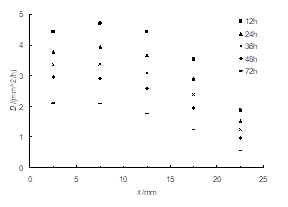Fig. 3. Variation of moisture diffusion coefficient as a function of space at specified time at Td=70 oC in experiment 1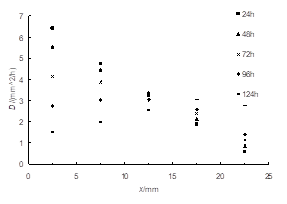Fig. 4. Variation of moisture diffusion coefficient as a function of space at specified time at Td=70 oC in experiment 2

The so-called representative moisture diffusion coefficient was obtained by averaging diffusion coefficient data at discrete points and specified times. Variations of representative moisture diffusion coefficient as a function of moisture content in different temperature for two experiments are presented in Figs. 5, 6, and 7. As moisture content decreased, representative moisture diffusion coefficient reduced, e.g., for the experiment 1 in Fig. 5 D were 3.0 mm2/h and 1.1 mm2/h when moisture content were 28.5% and 23.3%, respectively.

Wood temperature reached steady state rapidly when Td changed in terms of assumption 5, and the non-isothermal effect could be neglected. As wood temperature increased with increasing Td, the representative moisture diffusion coefficient increased, and the utmost values of D were 3.0 mm2/h, 4.8 mm2/h, 6.4 mm2/h at Td were 65 oC, 70 oC and 75 oC, respectively. This is due to diffusivity increasing as moisture gains more activation energy at higher temperature. Moreover, the effect of temperature on diffusion coefficient is more significant than that of moisture content.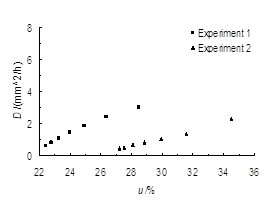Fig. 5. Variations of moisturediffusion coefficient with moisture content at Td =65 oC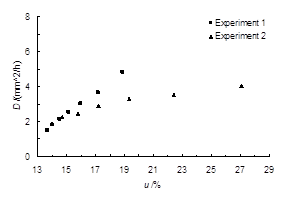Fig. 6. Variations of moisturediffusion coefficient with  moisture content at Td =70 oC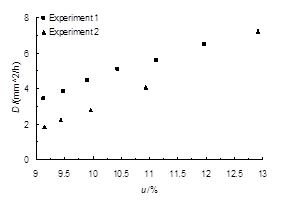Fig. 7. Variations of moisture diffusion coefficient with moisture content at Td =75 oC

The representative moisture diffusion coefficient in experiment 1 (D1) was larger than that in experiment 2 (D2) as moisture content was constant in Figs. 5, 6, and 7, e.g., D1=3.7 mm2/h, D2=2.9 mm2/h at 17.2% moisture content in Fig. 6. Wood is an anisotropic material, such that physical properties between different wood species, different specimen, different part of wood, such as early and late wood, sapwood and heartwood, are distinctive. Different specimens were used in two experiments and their diffusivity was different; as a result, it is not surprising that their moisture diffusion coefficients were different.

CONCLUSIONS

This paper presented results of moisture diffusion coefficients for Dahurian Larch board during convective drying by solving the Fick’s one-dimensional unsteady-state diffusion equation with the finite difference method after moisture content distributions at discrete points and specified time were obtained experimentally. From this investigation, the following conclusions can be drawn:

1. Generally speaking, the moisture diffusion coefficient decreased from the surface to the center of the Dahurian Larch wood.
2. Moisture diffusion coefficient decreased with the decreasing moisture content as the wood temperature was constant.
3. As wood temperature increased, the moisture diffusion coefficient increased, and the effect of wood temperature on moisture diffusion coefficient was more significant than that of moisture content.
4. Moisture diffusion coefficients were different for two experiments due to differences in the diffusivity properties of the specimens.

ACKNOWLEDGEMENT

This project was supported by the Innovation Program for Postgraduate of Northeast Forestry University (GRAM 09), the National Natural Science Fund of China (No. 30972306), and the Program 948 of State Forestry Bureau (No. 2006-4-105). The authors express their sincere gratitude for these funders.

REFERENCES CITED

Avramidis, S., and Siau, J. F. (1987). “An investigation of the external and internal resistance to moisture diffusion in wood,” Wood Sci. Technol. 21, 249-256.

Choong, E. T., and Skaar, C. (1969). “Separating internal and external resistance to moisture removal in wood drying,” J. Wood Science. 1(4), 200-202.

Liu, J. Y. (1989). “A new method for separating diffusion coefficient and surface emission coefficient,” Wood Fiber Sci. 21(2), 133-141.

Liu, J. Y., and Simpson, W. T. (1999). “Two-stage moisture diffusion in wood with constant transport coefficients,” Drying Tech. 17(1&2), 257-269.

Liu, J. Y., Simpson, W. T., and Verrill, S. P. (2001). “An inverse moisture diffusion algorithm for the determination of moisture diffusion,” Drying Tech. 19(8), 1555-1568.

Newman, A. B. (1931). “The drying of porous solids: Diffusion and surface-emission equations and diffusion calculations,” Trans. Am. Inst. Chem. Eng. 27, 203-211 and 310-333.

Skaar, C. (1954). “Analysis of methods for determining the coefficient of moisture diffusion in wood,” J. Forest. Prod. 4(6), 403-410.

Article submitted: November 23, 2010; Peer review completed: February 13, 2011; Revised version received: February 16, 2011; Accepted: February 20, 2011; Published: February 22, 2011.